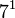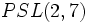# Groups of order 168

Jump to: navigation, search

## Contents

This article gives information about, and links to more details on, groups of order 168
See pages on algebraic structures of order 168| See pages on groups of a particular order

## Statistics at a glance

### Factorization and useful forms

The number 168 has prime factors 2, 3, and 7, with prime factorization:$\! 168 = 2^3 \cdot 3 \cdot 7 = 8 \cdot 3 \cdot 7$

Other expressions for this number are:$\! 168 = (7^3 - 7)/2 = 2^3(2^3 - 1)(2^3 - 2) = 84(3 - 1)$

### Group counts

Quantity Value List/comment
Total number of groups up to isomorphism 57
Number of abelian groups (i.e., finite abelian groups) up to isomorphism 3 (number of abelian groups of order$2^3$) times (number of abelian groups of order$3^1$) times (number of abelian groups of order$7^1$) =$3 \times 1 \times 1 = 3$. See classification of finite abelian groups and structure theorem for finitely generated abelian groups.
Number of nilpotent groups (i.e., finite nilpotent group)s up to isomorphism 5 (number of groups of order 8) times (number of groups of order 3) times (number of groups of order 7) =$5 \times 1 \times 1 = 5$. See number of nilpotent groups equals product of number of groups of order each maximal prime power divisor, which in turn follows from equivalence of definitions of finite nilpotent group.
Number of solvable groups (i.e., finite solvable group)s up to isomorphism 56 See note on non-solvable groups
Number of non-solvable groups up to isomorphism 1 The only non-solvable group is the simple non-abelian group projective special linear group:PSL(3,2), which is also isomorphic to$PSL(2,7)$.
Number of simple groups up to isomorphism (since the order is not prime, these are simple non-abelian groups) 1 the simple non-abelian group projective special linear group:PSL(3,2), which is also isomorphic to$PSL(2,7)$.
Number of quasisimple groups up to isomorphism 1 PSL(3,2)
Number of almost simple groups up to isomorphism 1 PSL(3,2)
Number of almost quasisimple groups up to isomorphism 1 PSL(3,2)
Number of perfect groups up to isomorphism 1 PSL(3,2)

## GAP implementation

The order 168 is part of GAP's SmallGroup library. Hence, any group of order 168 can be constructed using the SmallGroup function by specifying its group ID. Also, IdGroup is available, so the group ID of any group of this order can be queried.

Further, the collection of all groups of order 168 can be accessed as a list using GAP's AllSmallGroups function.

Here is GAP's summary information about how it stores groups of this order, accessed using GAP's SmallGroupsInformation function:

gap> SmallGroupsInformation(168);

There are 57 groups of order 168.
They are sorted by their Frattini factors.
1 has Frattini factor [ 42, 1 ].
2 has Frattini factor [ 42, 2 ].
3 has Frattini factor [ 42, 3 ].
4 has Frattini factor [ 42, 4 ].
5 has Frattini factor [ 42, 5 ].
6 has Frattini factor [ 42, 6 ].
7 - 11 have Frattini factor [ 84, 7 ].
12 - 18 have Frattini factor [ 84, 8 ].
19 - 21 have Frattini factor [ 84, 9 ].
22 has Frattini factor [ 84, 10 ].
23 has Frattini factor [ 84, 11 ].
24 - 28 have Frattini factor [ 84, 12 ].
29 - 33 have Frattini factor [ 84, 13 ].
34 - 38 have Frattini factor [ 84, 14 ].
39 - 41 have Frattini factor [ 84, 15 ].
42 - 57 have trivial Frattini subgroup.

For the selection functions the values of the following attributes
are precomputed and stored:
IsAbelian, IsNilpotentGroup, IsSupersolvableGroup, IsSolvableGroup,
LGLength, FrattinifactorSize and FrattinifactorId.

This size belongs to layer 2 of the SmallGroups library.
IdSmallGroup is available for this size.